## Symbol table

### applications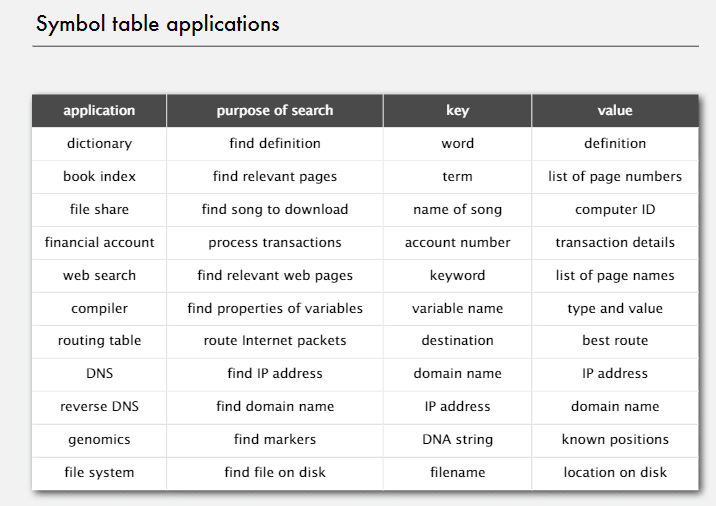### api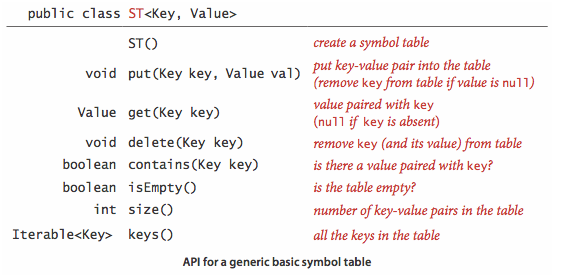• key is not null
• if key is not exist, get() will return null
• pull() will cover old value

### key and value

Value

Key

• Key是可比較的，即是`Comparable`的，並且使用`compareTo()`函數
• Key是泛型的
• 能用`equals()`判斷相等，能用`hashCode()`獲取Key（這兩個函數都是Java的內置函數）
• 對於Key最好是使用不可變的類型（`Integer``Double``String`之類的）

• 自反性：`x.equals(x)` is true
• 對稱性：`x.equals(y)` is true if and only if `y.equals(x)` is true
• 傳遞性：if `x.equals(y)` and `y.equals(z)` are true, then so is `x.equals(z)`
• 不為`null``x.equals(null)` return false

``````public class Date implements Comparable<Date> {
private final int month;
private final int day;
private final int year;

public Date(int month, int day, int year) {
this.month = month;
this.day = day;
this.year = year;
}

public boolean equals(Date that) {
if (this.day   != that.day  ) return false;
if (this.month != that.month) return false;
if (this.year  != that.year ) return false;
return true;
}
}
``````

• `final`字段，不然可能違反對稱性（繼承）
• 最好用`Object`（不過這個專家們目前還在激烈爭論中）
• 加入自反性，不為`null`的判斷，並且驗證類型一致性
• 關於比較一個類中的不同變量：
• 如果比較的是一個原始類型，用`==`
• 如果比較的是一個對象，用`equals()`
• 如果比較的是一個數組，對`Arrays.equals(a, b)`而不是`a.equals(b)`
``````public boolean equals(Object y) {
if (y == this)                       return true;
if (y == null)                       return false;
if (y.getClass() != this.getClass()) return false;
Date that = (Date) y;
if (this.day   != that.day  ) return false;
if (this.month != that.month) return false;
if (this.year  != that.year ) return false;
return true;
}
``````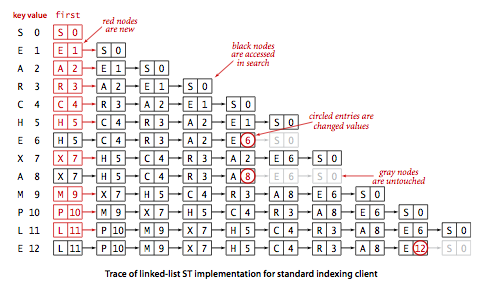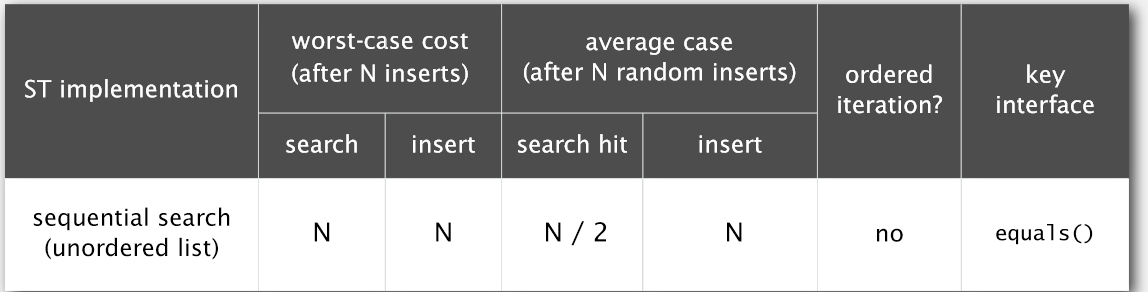## Ordered array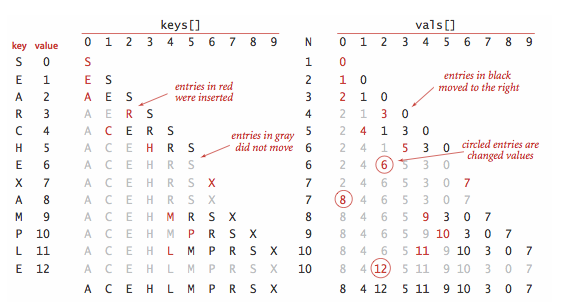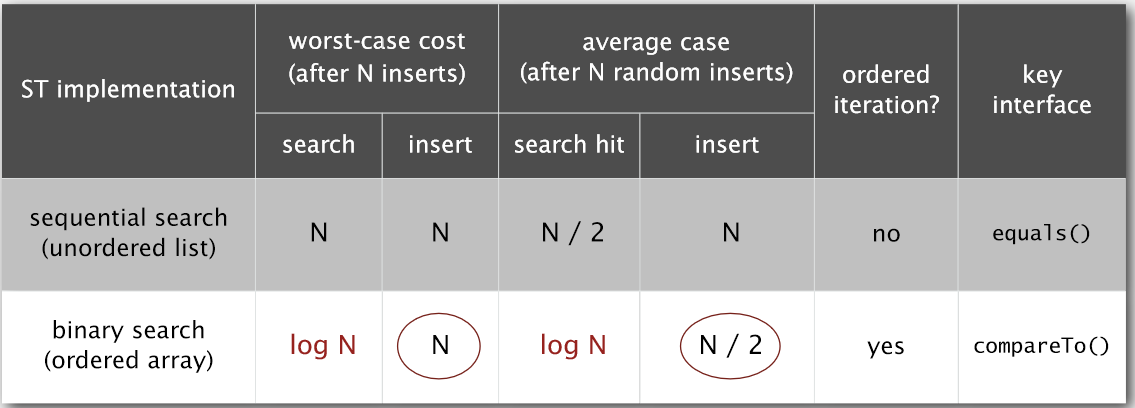## Ordered symbol tables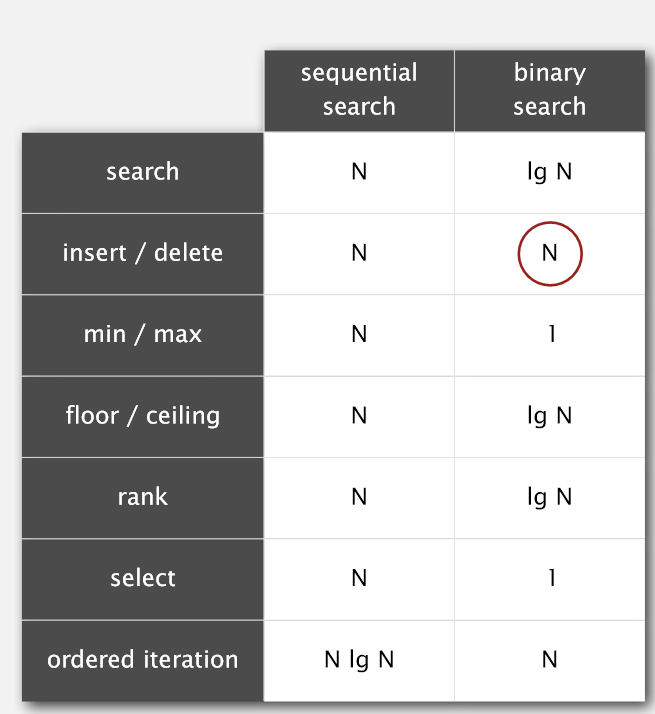Binary Search

• 如果search key < the key in the middle → 在子數組的左半部分搜索
• 如果search key > the key in the middle → 在子數組的右半部分搜索
• 否則the key in the middle == search key